### Real Vim ninjas count every keystroke - do you?

###### Pick a challenge, fire up Vim, and show us what you got.

Your VimGolf key: please sign in

$gem install vimgolf$ vimgolf setup
\$ vimgolf put 510a052c6db41b0002000028


### LaTeX to XML Math Delimiters

Vim is amazing when used to edit MediaWiki text, but typing "$. . .$" can be tiresome and frustrating if formulas are used often. LaTeX delimiters are so concise and even come in two flavors: "$$. . .$$" for inline math and "$. . .$" for centered formulas. The goal is to perform the following conversions: "$$. . .$$" becomes "$. . .$" "$. . .$" becomes "<center>$. . .$</center>"

##### Start file
Given two vectors $$\vec{x}$$ and $$\vec{y}$$ in $$\mathbb{R}^n$$,
their '''dot product''' or '''inner product''' is defined as the following:

$\sum_{i=0}^{n} x_i \, y_i$

----

Integration by parts is another way of writing the product rule of differentiation.
For two functions $$f(x)$$ and $$g(x)$$, the following are equivalent:

\begin{align} \frac{\mathrm{d}}{\mathrm{d}x} \left( f(x) \, g(x) \right) &= f'(x) \, g(x) + f(x) \, g'(x) \\ \int f(x) \, g'(x) \, \mathrm{d}x &= f(x) \, g(x) - \int f'(x) \, g(x) \, \mathrm{d}x \end{align}

----

Matrix multiplication is not commutative

\begin{align} \begin{bmatrix} a_{11} & a_{12} \\ a_{21} & a_{22} \end{bmatrix} \, \begin{bmatrix} b_{11} & b_{12} \\ b_{21} & b_{22} \end{bmatrix} &\ne \begin{bmatrix} b_{11} & b_{12} \\ b_{21} & b_{22} \end{bmatrix} \, \begin{bmatrix} a_{11} & a_{12} \\ a_{21} & a_{22} \end{bmatrix} \\ \begin{bmatrix} a_{11} \, b_{11} + a_{12} \, b_{21} & a_{11} \, b_{12} + a_{12} \, b_{22} \\ a_{21} \, b_{11} + a_{22} \, b_{21} & a_{21} \, b_{12} + a_{22} \, b_{22} \end{bmatrix} &\ne \begin{bmatrix} a_{11} \, b_{11} + a_{21} \, b_{12} & a_{12} \, b_{11} + a_{22} \, b_{12} \\ a_{11} \, b_{21} + a_{21} \, b_{22} & a_{12} \, b_{21} + a_{22} \, b_{22} \end{bmatrix} \begin{align}

''Quod erat demonstrandum''.

##### End file
Given two vectors $\vec{x}$ and $\vec{y}$ in $\mathbb{R}^n$,
their '''dot product''' or '''inner product''' is defined as the following:

<center>$\sum_{i=0}^{n} x_i \, y_i$</center>

----

Integration by parts is another way of writing the product rule of differentiation.
For two functions $f(x)$ and $g(x)$, the following are equivalent:

<center>\begin{align} \frac{\mathrm{d}}{\mathrm{d}x} \left( f(x) \, g(x) \right) &= f'(x) \, g(x) + f(x) \, g'(x) \\ \int f(x) \, g'(x) \, \mathrm{d}x &= f(x) \, g(x) - \int f'(x) \, g(x) \, \mathrm{d}x \end{align}</center>

----

Matrix multiplication is not commutative

\begin{align} \begin{bmatrix} a_{11} & a_{12} \\ a_{21} & a_{22} \end{bmatrix} \, \begin{bmatrix} b_{11} & b_{12} \\ b_{21} & b_{22} \end{bmatrix} &\ne \begin{bmatrix} b_{11} & b_{12} \\ b_{21} & b_{22} \end{bmatrix} \, \begin{bmatrix} a_{11} & a_{12} \\ a_{21} & a_{22} \end{bmatrix} \\ \begin{bmatrix} a_{11} \, b_{11} + a_{12} \, b_{21} & a_{11} \, b_{12} + a_{12} \, b_{22} \\ a_{21} \, b_{11} + a_{22} \, b_{21} & a_{21} \, b_{12} + a_{22} \, b_{22} \end{bmatrix} &\ne \begin{bmatrix} a_{11} \, b_{11} + a_{21} \, b_{12} & a_{12} \, b_{11} + a_{22} \, b_{12} \\ a_{11} \, b_{21} + a_{21} \, b_{22} & a_{12} \, b_{21} + a_{22} \, b_{22} \end{bmatrix} \begin{align}

''Quod erat demonstrandum''.


#### View Diff

1c1
< Given two vectors $$\vec{x}$$ and $$\vec{y}$$ in $$\mathbb{R}^n$$,
---
> Given two vectors $\vec{x}$ and $\vec{y}$ in $\mathbb{R}^n$,
4c4
< $\sum_{i=0}^{n} x_i \, y_i$
---
> <center>$\sum_{i=0}^{n} x_i \, y_i$</center>
9c9
< For two functions $$f(x)$$ and $$g(x)$$, the following are equivalent:
---
> For two functions $f(x)$ and $g(x)$, the following are equivalent:
11c11
< \begin{align} --- > <center>\begin{align} 14c14 < \end{align} --- > \end{align}</center>
20c20
< $$--- > $48c48 <$$ --- >$


### Solutions

The best way to learn is to practice. Below, you will find some of the solutions other golfers have entered. To unlock higher ranked solutions, submit your own entry which does as well or better than the solutions you can currently see - climb the ladder!

Check out these helpful resources to improve your Vim skills... Game on.

Unlock 17 remaining solutions by signing in and submitting your own entry
###### #18 David Wales / @selawdivad - Score: 144 - 02/04/13 @ 05:41
:%s/\$$/[itex]/g<CR>:%s/\$$/<\/math>/g<CR>:%s/\\$/<e<BS>center>[itex]/g<CR>:%s/\\$/<\/math><\/center>/g<CR>:%s/[itex] /[itex]/g<CR>:%s/ <\/math>/<\/math>/g<CR>:wq<CR>


## 18 active golfers, 40 entries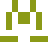63
###### #1 - Urtica dioica / @udioica

02/06/2013 at 11:40PM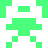75
###### #2 - Miłosz Łakomy / @foobar01123

12/28/2019 at 02:12PM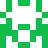82
###### #3 - Trevor Powell / @DoomedBunnies

02/05/2013 at 05:57AM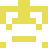82
###### #4 - sweet.mike.vg / @SweetMikeVg

05/02/2013 at 05:35AM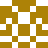86
###### #5 - lubyk / @lubyk_

05/21/2013 at 07:08AM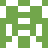87
###### #6 - Christopher Harrison / @Xophmeister

01/31/2013 at 06:37PM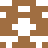89
###### #7 - Carlos A Henríquez Q / @lagunex

01/19/2015 at 02:48PM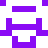90
###### #8 - mnx / @mnxx

01/31/2013 at 01:10PM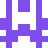90
###### #9 - matthieu le grix / @mlegrix

05/01/2015 at 08:10AM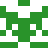91
###### #10 - hiding / @I_haveno_name

10/22/2013 at 01:12PM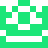92
###### #11 - Vim Golfer / @vimgolfern00b

01/31/2013 at 01:01PM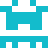92
###### #12 - Steve Tjoa / @stevetjoa

02/01/2013 at 10:35PM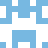94
###### #13 - Zach Kelling / @zeekay

02/25/2013 at 09:02AM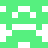95
###### #14 - Gareth Lloyd / @IgnitionWeb

03/08/2015 at 04:30PM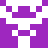98
###### #15 - Satoshi Kawasaki / @hobbes3k

04/18/2013 at 10:19PM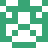99

08/22/2020 at 02:05AM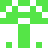118
###### #17 - Olivier Huber / @xhub

04/05/2013 at 10:55PM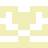144
###### #18 - David Wales / @selawdivad

02/04/2013 at 05:41AM